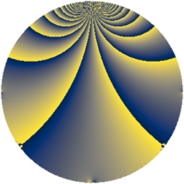# Properties

 Label 64.2.iLevel $64$ Weight $2$ Character orbit 64.i Rep. character $\chi_{64}(5,\cdot)$ Character field $\Q(\zeta_{16})$ Dimension $56$ Newform subspaces $1$ Sturm bound $16$ Trace bound $0$

# Related objects

## Defining parameters

 Level: $$N$$ $$=$$ $$64 = 2^{6}$$ Weight: $$k$$ $$=$$ $$2$$ Character orbit: $$[\chi]$$ $$=$$ 64.i (of order $$16$$ and degree $$8$$) Character conductor: $$\operatorname{cond}(\chi)$$ $$=$$ $$64$$ Character field: $$\Q(\zeta_{16})$$ Newform subspaces: $$1$$ Sturm bound: $$16$$ Trace bound: $$0$$

## Dimensions

The following table gives the dimensions of various subspaces of $$M_{2}(64, [\chi])$$.

Total New Old
Modular forms 72 72 0
Cusp forms 56 56 0
Eisenstein series 16 16 0

## Trace form

 $$56 q - 8 q^{2} - 8 q^{3} - 8 q^{4} - 8 q^{5} - 8 q^{6} - 8 q^{7} - 8 q^{8} - 8 q^{9} + O(q^{10})$$ $$56 q - 8 q^{2} - 8 q^{3} - 8 q^{4} - 8 q^{5} - 8 q^{6} - 8 q^{7} - 8 q^{8} - 8 q^{9} - 8 q^{10} - 8 q^{11} - 8 q^{12} - 8 q^{13} - 8 q^{14} - 8 q^{15} - 8 q^{16} - 8 q^{17} - 8 q^{18} - 8 q^{19} - 8 q^{20} - 8 q^{21} - 8 q^{23} + 32 q^{24} - 8 q^{25} + 32 q^{26} - 8 q^{27} + 32 q^{28} - 8 q^{29} + 72 q^{30} + 32 q^{32} + 32 q^{34} - 8 q^{35} + 72 q^{36} - 8 q^{37} + 32 q^{38} - 8 q^{39} + 32 q^{40} - 8 q^{41} + 32 q^{42} - 8 q^{43} - 8 q^{45} - 8 q^{46} - 8 q^{47} - 8 q^{48} - 8 q^{49} - 32 q^{50} + 24 q^{51} - 56 q^{52} - 8 q^{53} - 72 q^{54} + 56 q^{55} - 64 q^{56} - 8 q^{57} - 80 q^{58} + 56 q^{59} - 104 q^{60} - 8 q^{61} - 40 q^{62} + 64 q^{63} - 104 q^{64} - 16 q^{65} - 88 q^{66} + 72 q^{67} - 56 q^{68} - 8 q^{69} - 104 q^{70} + 56 q^{71} - 80 q^{72} - 8 q^{73} - 64 q^{74} + 56 q^{75} - 72 q^{76} - 8 q^{77} - 32 q^{78} + 24 q^{79} + 32 q^{80} - 8 q^{81} + 72 q^{82} - 8 q^{83} + 104 q^{84} - 8 q^{85} + 96 q^{86} - 8 q^{87} + 72 q^{88} - 8 q^{89} + 136 q^{90} - 8 q^{91} + 144 q^{92} + 16 q^{93} + 88 q^{94} + 128 q^{96} + 128 q^{98} + 16 q^{99} + O(q^{100})$$

## Decomposition of $$S_{2}^{\mathrm{new}}(64, [\chi])$$ into newform subspaces

Label Dim $A$ Field CM Traces $q$-expansion
$a_{2}$ $a_{3}$ $a_{5}$ $a_{7}$
64.2.i.a $56$ $0.511$ None $$-8$$ $$-8$$ $$-8$$ $$-8$$